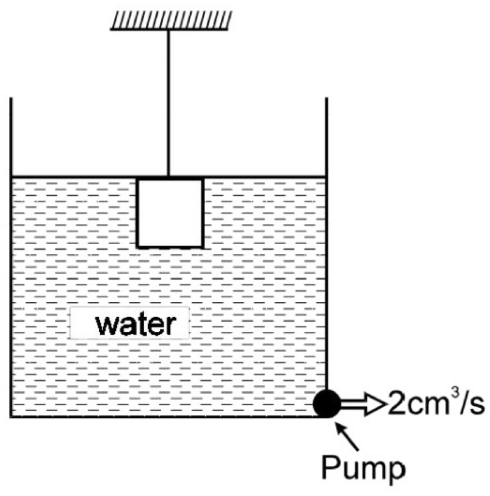# Fluid mechanicsThe figure above shows a cubical block of side $10\text{ cm}$ and relative density 1.5 suspended by a wire of cross-sectional area $10^{-6} \text{ m}^2$. The breaking stress of the wire is $7\times 10^6 \text{ N/m}^2$. The block is placed in a beaker of base area $200\text{ cm}^2$ and initially i.e. at $t=0$, the top surface of the water and the block coincide. There is a pump at the bottom corner which ejects $2\text{ cm}^3$ of water per second. Find the time at which the wire will break.

Take $g = 10\text{ m/s}^2$.

×